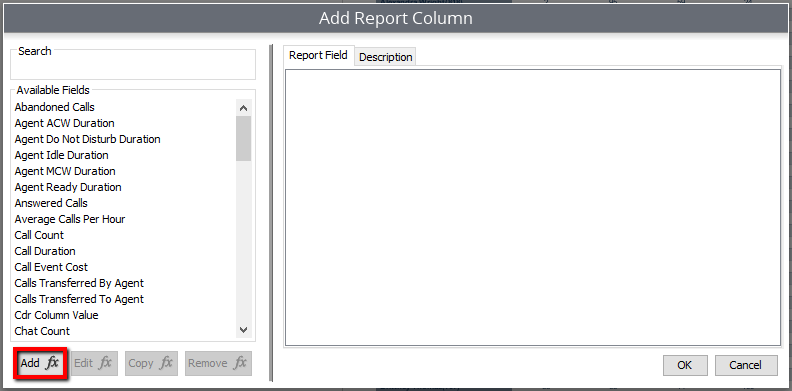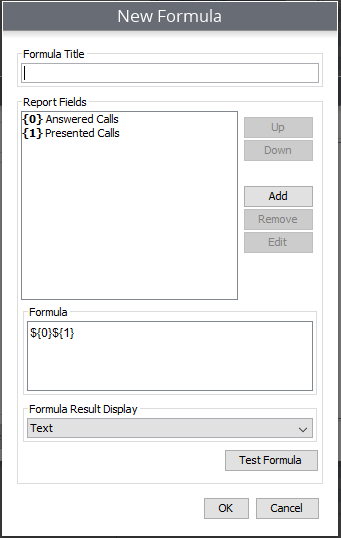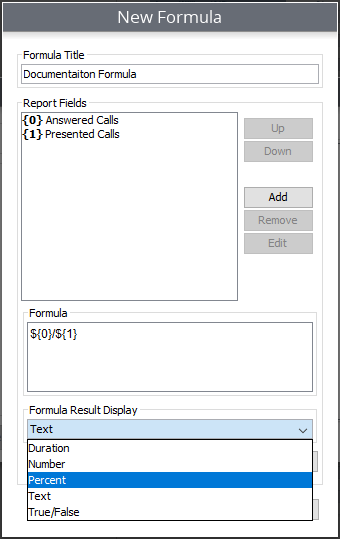# Creating Custom Formulas

This provides instructions on how to create custom formulas for a custom report. Access to this feature requires the Custom Reports module.

When creating and editing a report you are provided with complete flexibility. This means that you can either use the provided metrics as is, or you can adjust them to fit your needs.

However, if you are not able to find the metric that fits your needs, or if you want to provide further depth to your metrics, you will also have the option to create your own formulas. This is done by selecting metrics combined with simple mathematical equations.

1. While creating or editing a report select "Add" to add a new column to the report.
2. Select the "Add Formula" button.# Selecting the Metrics

The "New Formula" window will appear and you can now begin to build your formula.

1. Start by naming your formula.
2. Now you can select the metrics that you will use to build your formula. Start by hitting "Add" on the right-hand side. You will now be presented with a window displaying the metric options available for you to choose from.
3. Select the desired metric, then select "OK."

Repeat steps 2 and 3 until you have selected the metrics necessary to build your formula.

# Building the Formula

Once you have selected the desired metrics, you can now build your formula.

In the Formula window you will see the metrics that you have selected represented by a dollar sign and curly brackets surrounding a number (\${0}). The dollar sign signifies the beginning of a metric and the number coincides with the metric selected in the Report Fields section above.To create the formula, we will now have to create the mathematical equation. In this scenario we want to see a percentage of calls answered compared to calls presented. In the formula window, you would see \${0}\${1}. To achieve the desired result, a division symbol will be placed between the two metrics.

It would look like this \${0}/\${1}.

Once the formula is complete, select the Formula Result Display drop down, and select the desired result display.(i.e., duration, number, percent, etc.)You can also select Test Formula. This will assist in verifying that your equation makes sense.

Once your formula is complete, it will now be featured in your list of metric options, meaning it can be used on future reports as well.